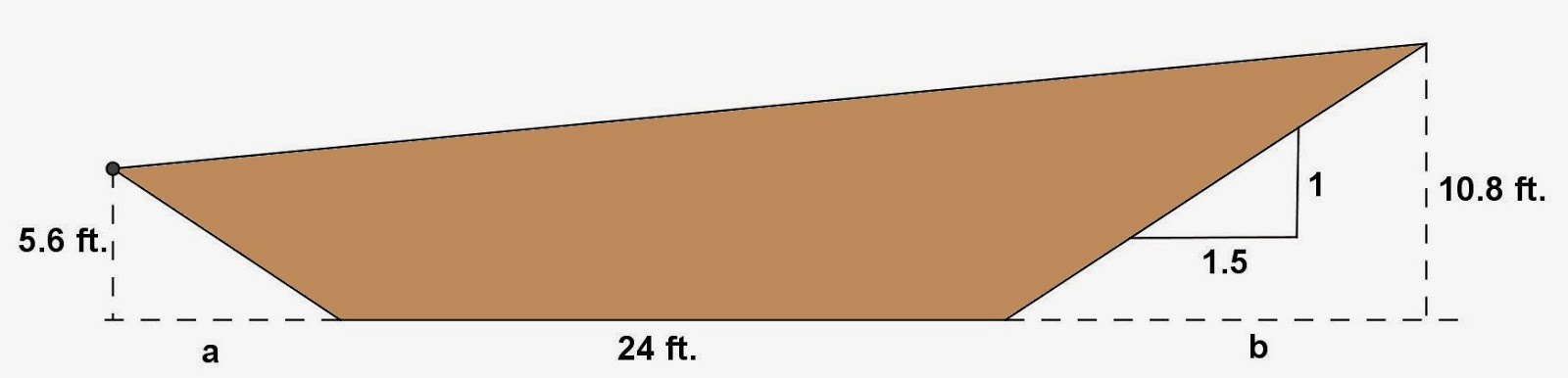Showing posts with label Solid Geometry. Show all posts
Showing posts with label Solid Geometry. Show all posts

## Sunday, March 1, 2015

### Circular Cylinder Problems, 8

Category: Solid Geometry

"Published in Newark, California, USA"

The plumb-line distance from the lowest point of the top of the Leaning Tower of Pisa to the ground is 179 ft. The tower leans so that this plumb line strikes the ground 14 ft. from the nearest point of the base as shown. (a) At what angle is its axis now inclined from the vertical? (b) At what angle would its axis have to be inclined from the vertical before it would topple over?Photo by Math Principles in Everyday Life

Solution:

(a) To understand more the problem, let's label further the figure as followsPhoto by Math Principles in Everyday Life

By Pythagorean Theorem, the length of the Leaning Tower of Pisa is

Therefore, the angle of inclination with respect to vertical axis for the Leaning Tower of Pisa is

or

(b) If the Leaning Tower of Pisa is inclined from the vertical before it would topple over, then the figure will be like thisPhoto by Math Principles in Everyday Life

Therefore, the angle of inclination with respect to vertical axis for the Leaning Tower of Pisa before it would topple over is

or

## Saturday, February 28, 2015

### Rectangular Parallelepiped Problems, 17

Category: Solid Geometry

"Published in Newark, California, USA"

Find the angles that the diagonal of a rectangular parallelepiped 1 in. by 3 in. by 5 in. make with the faces.

Solution:

To illustrate the problem, it is better to draw the figure as followsPhoto by Math Principles in Everyday Life

Next, we need to draw a diagonal line at the top and bottom of the rectangular parallelepiped as well as its diagonal and angles as followsPhoto by Math Principles in Everyday Life

By using Pythagorean Theorem, the length of d is

Therefore, the value of an angle is

or

Next, we need to draw a diagonal line at the left and right of the rectangular parallelepiped as well as its diagonal and angles as followsPhoto by Math Principles in Everyday Life

Therefore, the value of an angle is

or

Next, we need to draw a diagonal line at the front and back of the rectangular parallelepiped as well as its diagonal and angles as followsPhoto by Math Principles in Everyday Life

Therefore, the value of an angle is

or

## Friday, February 27, 2015

### Trapezoid Prism Problems, 8

Category: Solid Geometry

"Published in Newark, California, USA"

The figure shows a right section of a railroad cut in a hillside. If the sides rise 1 unit vertically to a horizontal distance of 1.5 units, and if the length of the cut is 100 ft., find the volume of earth removed.Photo by Math Principles in Everyday Life

Solution:

The cross section of a railroad cut in a hillside is a general quadrilateral. If you draw vertical lines from the top ends of a general quadrilateral to the bottom horizontal line that contains one side, then it becomes a trapezoid as followsPhoto by Math Principles in Everyday Life

By similar triangles, we can solve for the values of a and b as follows

The area of a trapezoid is

The area of small triangle is

The area of big triangle is

Hence, the area of the base which is the cross section of a railroad cut in a hillside is

Therefore, the volume of earth removed which is the volume of a prism is